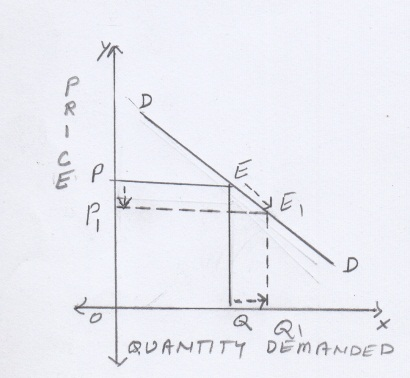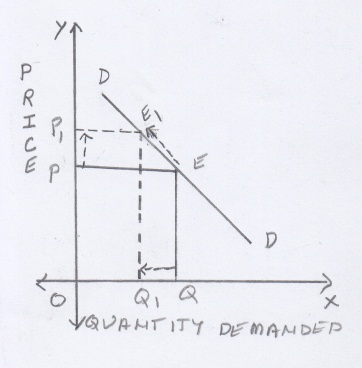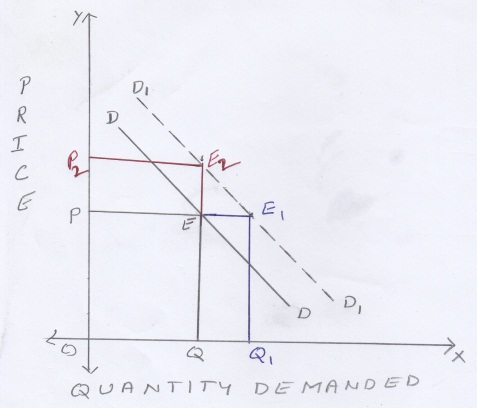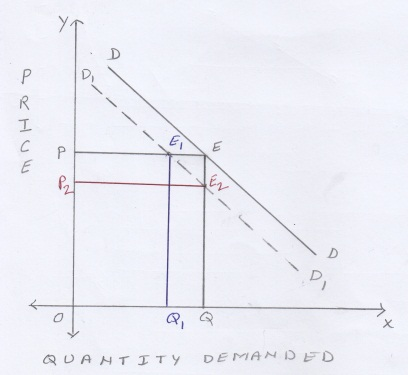# SHIFTS IN DEMAND

 VARIATIONS–IN-DEMAND CHANGES-IN-DEMAND Variations in demand take place because of a change in price.. **Changes in demand take place because of a change in factors other than price Extension in demand Contraction in demand Increase in demand Decrease in demandPrice is measured on the Y axis; quantity demanded is measured on the X axis; OP is the original price of the commodity; OQ is the original quantity demanded and E is the original equilibrium point on the original demand curve DD Variations in demand are shown along the same demand curve DD. Demand curve shifts to the right of the original, from DD to D1D1. Demand curve shifts to the left of the original from DD to D1D1 When the price falls from OP to OP1, quantity demanded extends from OQ to OQ1. Therefore this is known as extension in demand. When the price risess from OP to OP1, quantity demanded contracts from OQ to OQ1 Therefore this is known as contraction in demand a)At the same price PO more is demanded ie QO1 instead of OQb) The same quantity OQ is demanded at a higher price ie OP2 instead of OP a)At the same price PO less is demanded ie QO1 instead of OQb) The same quantity OQ is demanded at a lower price ie OP2 instead of OP The equilibrium point E shifts downwards to E1 on the same demand curve DD The equilibrium point E shifts upwards to E1 on the same demand curve DD The equilibrium point E shifts either to  E1 or toE2 on the new demand curve D1D1 to the right of the original demand curve DD The equilibrium point E shifts either to  E1 or toE2 on the new demand curve D1D1 to the left of the original demand curve DD

** Change in non- price ie factors influencing demand and assumptions of the law of demand.

Posted in General Economics